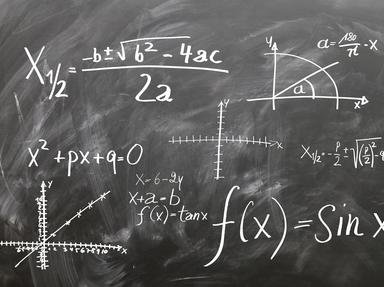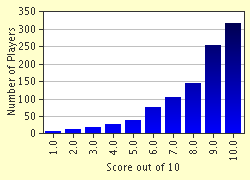# High School Level Maths [ 1 ] Trivia Quiz

### Try these without looking up any books or using a calculator or even a pen and pencil. Good Luck!

A multiple-choice quiz by LMN. Estimated time: 4 mins.

Author
LMN
Time
4 mins
Type
Multiple Choice
Quiz #
3,241
Updated
Dec 03 21
# Qns
10
Difficulty
Average
Avg Score
8 / 10
Plays
16473
Last 3 plays: IMACLINT (8/10), Guest 198 (7/10), Guest 74 (10/10).
This quiz has 2 formats: you can play it as a or as shown below.
Scroll down to the bottom for the answer key.
1. What is the number called located on the bottom part of a fraction? Hint

numerator
denominator
lower fraction
vinculum

#### NEXT>

2. What is 15% of \$200?

Answer: (Change 15% to a fraction)

#### NEXT>

3. Which of the following terms would you more likely come across while studying Trigonometry? Hint

Dollars
Binary
Skew Lines
Sine

#### NEXT>

4. Parallel lines ... Hint

eventually touch
cross only once
never meet
are never the same distance apart

#### NEXT>

5. Whose formula for triangles says that the square of the hypotenuse is equal to the sum of the squares of the other two sides? Hint

Plato
Pythagoras
Hypotenuse
Triangulas

#### NEXT>

6. Finish this sequence by writing in the next appropriate word ... triangle, quadrilateral, pentagon, __________ ?

#### NEXT>

7. The circumference of a circle can also be called the _________ Hint

area
perimeter
diameter

#### NEXT>

8. Solving the quadratic equation (x+1)(x-1)=0 will give you an answer of: Hint

x = 1
x = 0
x = -1
x = 1 , -1

#### NEXT>

9. What will applying the formula: 'Multiplying the base and the height and then dividing by two' give you an answer for? Hint

Area of a rectangle
Area of a triangle
Diagonal of a polygon
Hypotenuse

#### NEXT>

10. Which area of Mathematics deals with protractors, segments, circumferences and sectors? Hint

Consumer Arithmetic
Fractions
Geometry
Rates and Ratios

Quiz Answer Key and Fun Facts
1. What is the number called located on the bottom part of a fraction?

Numerator is on the top and Denominator is on the bottom.
2. What is 15% of \$200?

15% of 200 = 15/100 x 200 = 30
3. Which of the following terms would you more likely come across while studying Trigonometry?

Sine, Cosine and Tangent Ratios are the basis for Trigonometry taught in High School.
4. Parallel lines ...

Parallel lines never meet and are always the same distance apart.
5. Whose formula for triangles says that the square of the hypotenuse is equal to the sum of the squares of the other two sides?

Pythagoras' rule for right angled triangles: the square of the hypotenuse (the longest side) is equal to the sum of the squares of the other two sides. It always seems to work!
6. Finish this sequence by writing in the next appropriate word ... triangle, quadrilateral, pentagon, __________ ?

A hexagon is a 6 sided polygon.
7. The circumference of a circle can also be called the _________

Circumference is the outside length around the circle.
8. Solving the quadratic equation (x+1)(x-1)=0 will give you an answer of:

Answer: x = 1 , -1

(x+1)(x-1) is a quadratic and hence can have more than one correct answer. Positive and Negative 1 both apply.
9. What will applying the formula: 'Multiplying the base and the height and then dividing by two' give you an answer for?

10. Which area of Mathematics deals with protractors, segments, circumferences and sectors?

Geometry looks at the space within and around two dimensional and three dimensional objects.
Source: Author LMN

This quiz was reviewed by FunTrivia editor crisw before going online.
Any errors found in FunTrivia content are routinely corrected through our feedback system.
Most Recent Scores
Today : IMACLINT: 8/10
Jan 27 2023 : Guest 198: 7/10
Jan 24 2023 : Guest 74: 10/10
Jan 24 2023 : Guest 69: 6/10
Jan 24 2023 : davobwd9: 5/10
Jan 22 2023 : Guest 73: 9/10
Jan 22 2023 : Guest 176: 10/10
Jan 21 2023 : BLJEFAM: 10/10
Jan 21 2023 : Guest 143: 4/10

Score DistributionRelated Quizzes
This quiz is part of series This is so easy!:

I don't mind taking quizzes that are easy to complete. I don't think quizzes need to be challenging. In this list are twenty quizzes that I think are easy (not necessarily rated easy), one quiz for each category.

1. 15 Land Animals with 3 Letter Names Easier
2. NOT Days of the Strong Easier
3. Which Actor Am I? Very Easy
4. Let's learn our ABCs the Disney Way Easier
5. 25 Questions For Children Very Easy
6. Just Fill in the Correct Letter! Average
7. 9 for 10 - Peru Average
8. The Cold War Easier
9. Games We Love Easier
10. Which Is The Correct Spelling? Easier
11. Name the Author Average
12. "Star Trek" Subtitles Easier